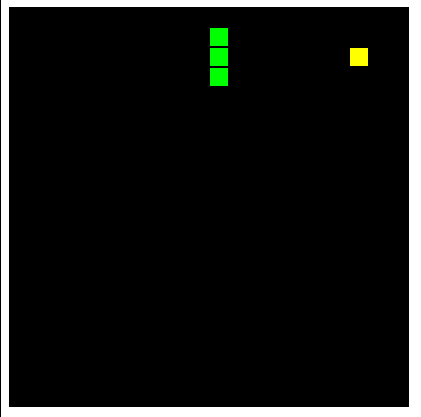tangloong2016-03-23 10:28:28

# js開發實現簡單貪吃蛇游戲（20行代碼）

```<!doctype html>
<html>
<body>
<canvas id="can" width="400" height="400" style="background:Black"></canvas>
<script>
var sn=[42,41],dz=43,fx=1,n,ctx=document.getElementById("can").getContext("2d");
function draw(t,c){
ctx.fillStyle=c;
ctx.fillRect(t%20*20+1,~~(t/20)*20+1,18,18);
}
document.onkeydown=function(e){fx=sn-sn==(n=[-1,-20,1,20][(e||event).keyCode-37]||fx)?fx:n};
!function(){
sn.unshift(n=sn+fx);
if(sn.indexOf(n,1)>0 || n<0||n>399||fx==1&&n%20==0||fx==-1&&n%20==19) return alert("GAME OVER");
draw(n,"Lime");
if(n==dz){
while(sn.indexOf(dz=~~(Math.random()*400))>=0);
draw(dz,"Yellow");
}else
draw(sn.pop(),"Black");
setTimeout(arguments.callee,130);
}();
</script>
</body>
</html>```gif動圖2個評論2個牛幣網頁游戲

• /
• /snakeat.html掃描二維碼關注最代碼為好友
2008大乐透走势图